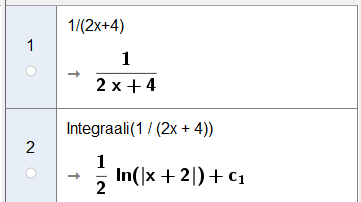# GeoGebra gives the wrong integral

Elias Heikkilä shared this question 1 year ago

Hello,

I'm experiencing problems with my GeoGebra. I opened CAS to define an integral: 1/(2x+4) and GeoGebra gave me 1/2ln(|x+2|), which is wrong.It is correct. 2 is a common factor of the terms at the denominator, so you can write it as 1/2(x+4).

The term 1/2 is a multiplicative constant, so you can pull it off the integral.

So your integral becomes 1/2 Integral(1/(x+2))dx.

Since the numerator is the derivative of the denominator, the integral is ln|denominator|, which is multiplied by coefficient 1/2.

Hence the solution is correct (and well simplified).2

It is correct. 2 is a common factor of the terms at the denominator, so you can write it as 1/2(x+4).

The term 1/2 is a multiplicative constant, so you can pull it off the integral.

So your integral becomes 1/2 Integral(1/(x+2))dx.

Since the numerator is the derivative of the denominator, the integral is ln|denominator|, which is multiplied by coefficient 1/2.

Hence the solution is correct (and well simplified).